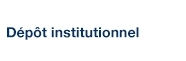# Approximation for Frobenius algebraic equations in Witt vectors

Bélair, Luc (2009). « Approximation for Frobenius algebraic equations in Witt vectors ». Journal of Algebra, 321(9), pp. 2353-2364.

Fichier(s) associé(s) à ce document :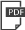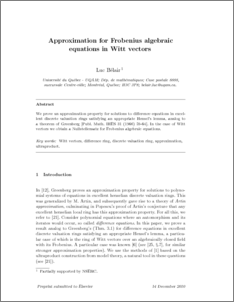Prévisualisation
PDF
Télécharger (223kB)

## Résumé

Abstract We prove an approximation property for solutions to difference equations in excellent discrete valuation rings satisfying an appropriate Hensel's lemma, analog to a theorem of Greenberg [M. Greenberg, Rational points in henselian discrete valuation rings, Publ. Math. Inst. Hautes Études Sci. 31 (1966) 59–64]. In the case of Witt vectors we obtain a Nullstellensatz for Frobenius algebraic equations.

Type: Article de revue scientifique Witt vectors; Difference ring; Discrete valuation ring; Approximation; Ultraproduct. Faculté des sciences > Département de mathématiques Luc Bélair 23 mars 2009 01 nov. 2014 02:08 http://archipel.uqam.ca/id/eprint/1804

## Statistiques

Voir les statistiques sur cinq ans...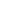RECHERCHER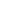PARCOURIR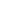LIBRE ACCÈS